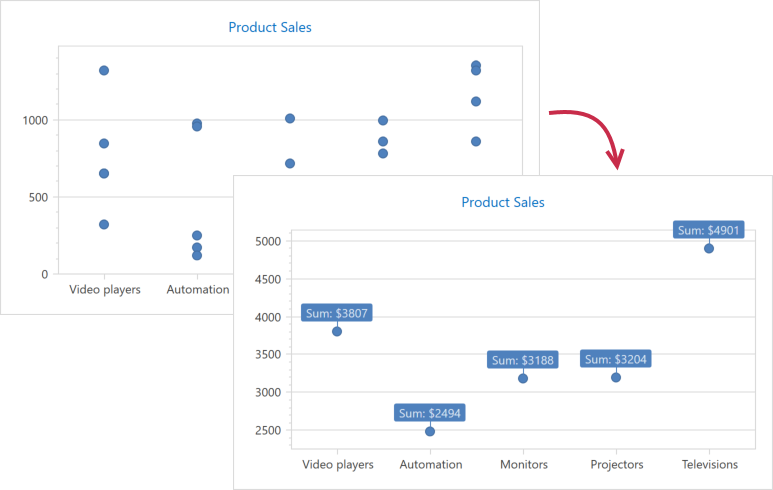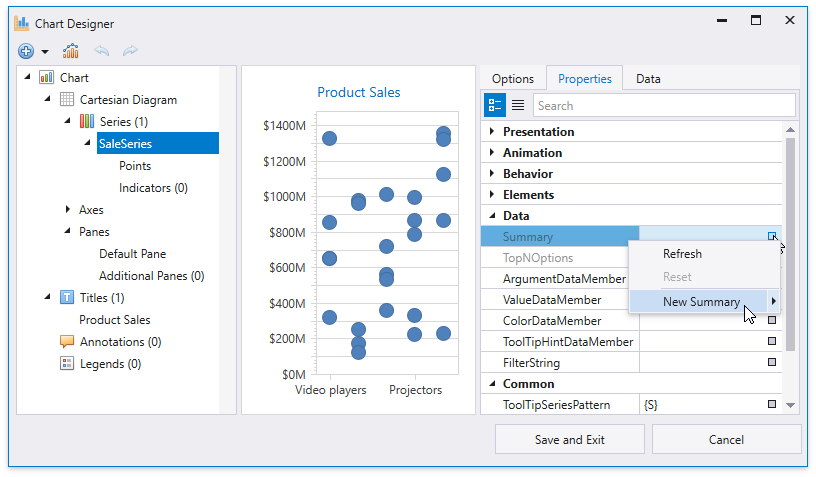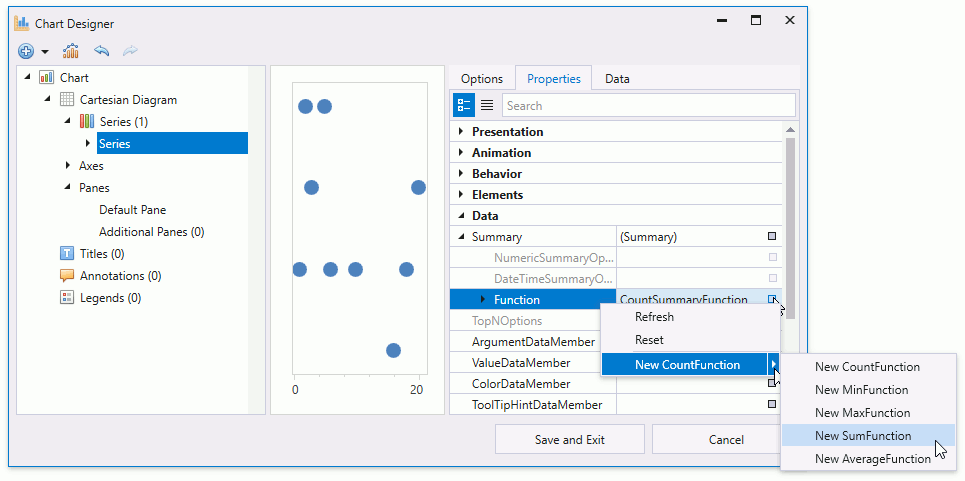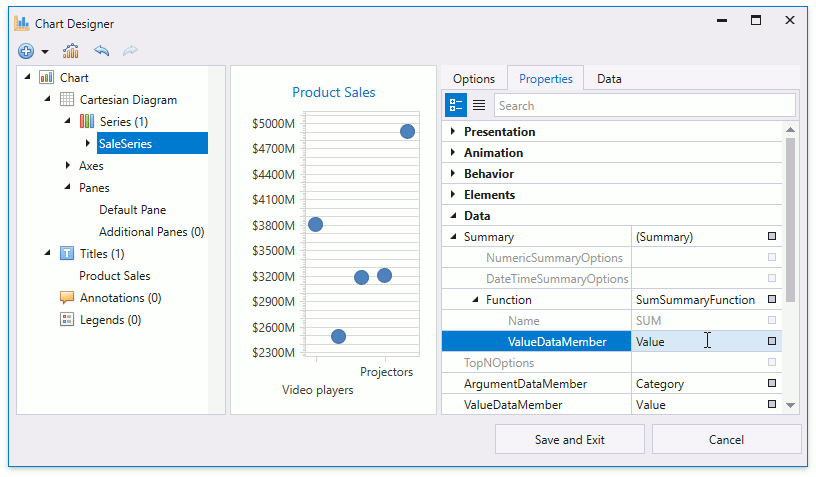# Calculate Summaries

The Chart Control can apply a summary function to calculate data source value summaries and display them as series points.## Summary vs Aggregation

Aggregation is similar to summaries but the Chart Control queries and stores data in memory differently.

Summary Aggregation
Store Data Chart stores summarized values. Chart stores raw data source values.
Change Detail Level Chart re-loads data from the data source. Chart re-calculates data in memory.
Behave on Zoom Chart does not re-calculate aggregated values when it is zoomed. Chart re-calculates value aggregates on each zoom level.
Memory Consumption Low High

## Apply a Summary Function

You can use Chart Designer or markup to apply a summary function.

Important

Automatic summary functions can be calculated only for Series that operate with data points with one value for each argument. If series data points have two or more values (e.g., Range, Financial and Bubble series), you should use Custom Summary Functions instead.

Also note that a summary function cannot be calculated when an axis’s scale mode is Continuous.

### Use the Chart Designer

• Run the Chart Designer.

• Use the Element Tree on the left to select a series. Then, open its Properties tab on the right.

• Click the Summary property’s button and select the New Summary item.• Use the Function property menu to select a summary function.• Type a name of the data source field that provides data for summarization.• Click the Save and Exit button to save changes and close the designer.

### Use Markup

The following example shows how to apply the “SUM” function:

``````<Window.DataContext>
<local:ChartViewModel/>
</Window.DataContext>
<Grid>
<dxc:ChartControl>
<dxc:XYDiagram2D SeriesItemsSource="{Binding SaleSeries}">
<dxc:XYDiagram2D.SeriesItemTemplate>
<DataTemplate>
<dxc:PointSeries2D DataSource="{Binding Values}"
ArgumentDataMember="Category">
<!-- Configure the summary function options. -->
<dxc:PointSeries2D.Summary>
<dxc:Summary>
<dxc:Summary.Function>
<dxc:SumSummaryFunction ValueDataMember="Value"/>
</dxc:Summary.Function>
</dxc:Summary>
</dxc:PointSeries2D.Summary>
<!--...-->
<dxc:PointSeries2D.Label>
<dxc:SeriesLabel Visible="True"
TextPattern="Sum: \${V}M"
dxc:MarkerSeries2D.Angle="90"
ResolveOverlappingMode="JustifyAroundPoint"/>
</dxc:PointSeries2D.Label>
</dxc:PointSeries2D>
</DataTemplate>
</dxc:XYDiagram2D.SeriesItemTemplate>
<!--...-->
</dxc:XYDiagram2D>
<!--...-->
</dxc:ChartControl>
</Grid>
``````

The example above uses the following API members to configure summary calculations:

Member Description
Series.Summary Gets or sets the series data point summarization settings.
Summary Stores the series data point summarize options.
Summary.Function Specifies the summary function that calculates data point values.
DataMemberSummaryFunction.ValueDataMember Gets or sets the data source field that provides data to be aggregated.

### Create a Custom Summary Function

The following example shows how to create the “Standard Deviation (STDEV.P)” summary function and apply it to chart data:

``````<Window.DataContext>
<local:ChartViewModel/>
</Window.DataContext>
<Grid>
<dxc:ChartControl>
<dxc:XYDiagram2D SeriesItemsSource="{Binding SaleSeries}">
<dxc:XYDiagram2D.SeriesItemTemplate>
<DataTemplate>
<dxc:PointSeries2D DataSource="{Binding Values}"
ArgumentDataMember="Category">
<!-- Configure the summary function options. -->
<dxc:PointSeries2D.Summary>
<dxc:Summary>
<dxc:Summary.Function>
<local:StdDevSummaryFunction ValueDataMember="Value"/>
</dxc:Summary.Function>
</dxc:Summary>
</dxc:PointSeries2D.Summary>
<!--...-->
</dxc:PointSeries2D>
</DataTemplate>
</dxc:XYDiagram2D.SeriesItemTemplate>
<!--...-->
</dxc:XYDiagram2D>
<!--...-->
</dxc:ChartControl>
</Grid>
``````

## Numeric, Time-Span and Date-Time Axis Specifics

For numerical, time-span and date-time x-axis scales, the summary function aggregates data point values for each interval the MeasureUnit (NumericSummaryOptions.MeasureUnit, TimeSpanSummaryOptions.MeasureUnit or DateTimeSummaryOptions.MeasureUnit) property specifies.

The following images illustrate how changes to the measurement unit affect point summaries:

The following example shows how to change a measurement unit used to calculate numeric x-axis arguments:

``````<Window.DataContext>
<local:ChartViewModel/>
</Window.DataContext>
<Grid>
<dxc:ChartControl>
<dxc:XYDiagram2D>
<dxc:PointSeries2D DataSource="{Binding Data}"
ArgumentDataMember="Argument">
<!-- Configure the summary function options. -->
<dxc:PointSeries2D.Summary>
<dxc:Summary>
<dxc:Summary.Function>
<dxc:AverageSummaryFunction ValueDataMember="Value"/>
</dxc:Summary.Function>
<dxc:Summary.NumericSummaryOptions>
<dxc:NumericSummaryOptions MeasureUnit="5"/>
</dxc:Summary.NumericSummaryOptions>
</dxc:Summary>
</dxc:PointSeries2D.Summary>
<!--...-->
</dxc:PointSeries2D>
</dxc:ChartControl>
</Grid>
``````